# http www comp nus edu sgcs 2100 Lecture

• Slides: 42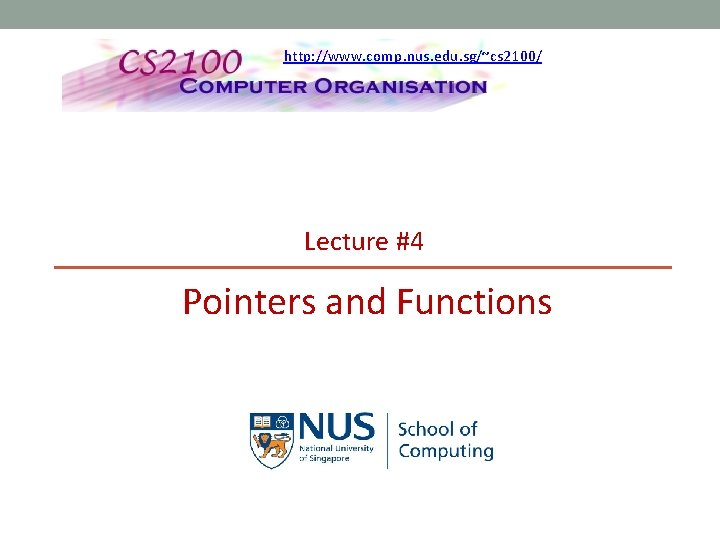http: //www. comp. nus. edu. sg/~cs 2100/ Lecture #4 Pointers and Functions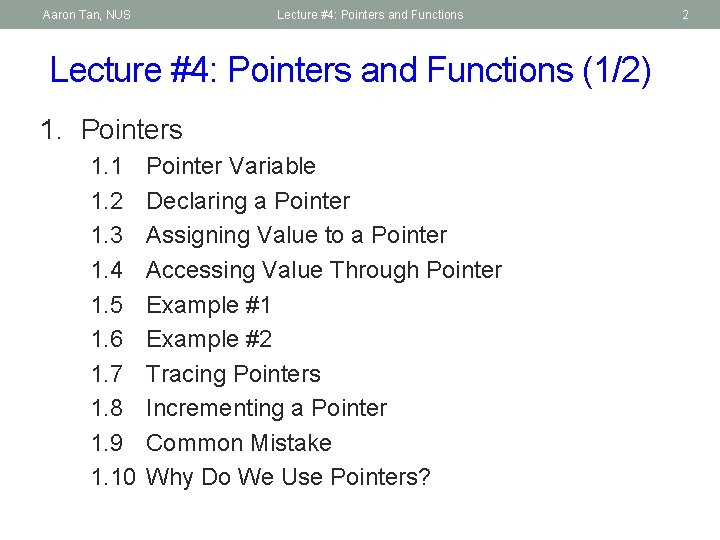Aaron Tan, NUS Lecture #4: Pointers and Functions (1/2) 1. Pointers 1. 1 1. 2 1. 3 1. 4 1. 5 1. 6 1. 7 1. 8 1. 9 1. 10 Pointer Variable Declaring a Pointer Assigning Value to a Pointer Accessing Value Through Pointer Example #1 Example #2 Tracing Pointers Incrementing a Pointer Common Mistake Why Do We Use Pointers? 2Aaron Tan, NUS Lecture #4: Pointers and Functions (2/2) 2. Calling Functions 3. User-Defined Functions 4. Pass-by-Value and Scope Rule 4. 1 Consequence of Pass-by-Value 5. Functions with Pointer Parameters 5. 1 Function to Swap Two Variables 5. 2 Examples 3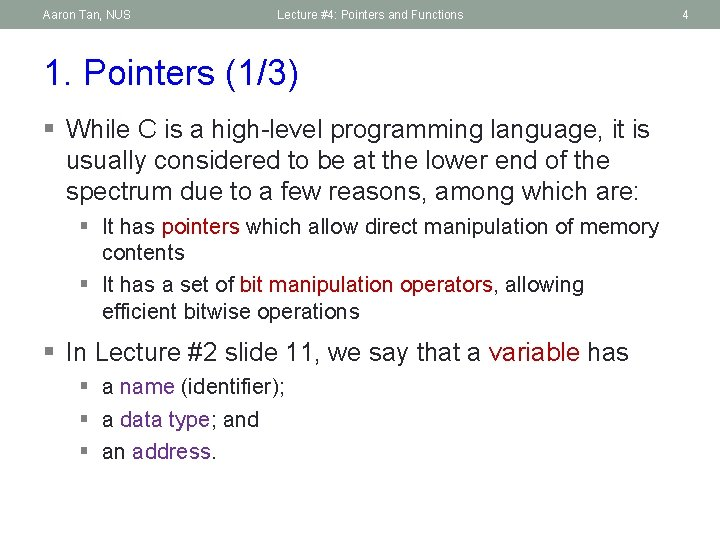Aaron Tan, NUS Lecture #4: Pointers and Functions 1. Pointers (1/3) § While C is a high-level programming language, it is usually considered to be at the lower end of the spectrum due to a few reasons, among which are: § It has pointers which allow direct manipulation of memory contents § It has a set of bit manipulation operators, allowing efficient bitwise operations § In Lecture #2 slide 11, we say that a variable has § a name (identifier); § a data type; and § an address. 4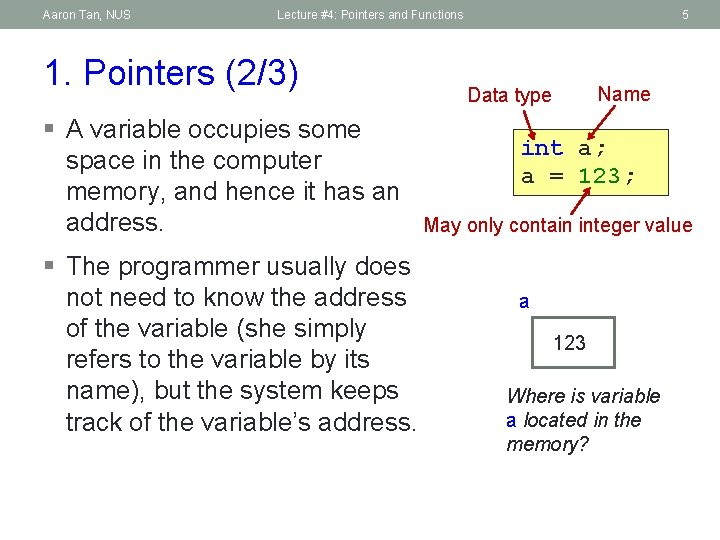Aaron Tan, NUS Lecture #4: Pointers and Functions 1. Pointers (2/3) § A variable occupies some space in the computer memory, and hence it has an address. § The programmer usually does not need to know the address of the variable (she simply refers to the variable by its name), but the system keeps track of the variable’s address. 5 Name Data type int a; a = 123; May only contain integer value a 123 Where is variable a located in the memory?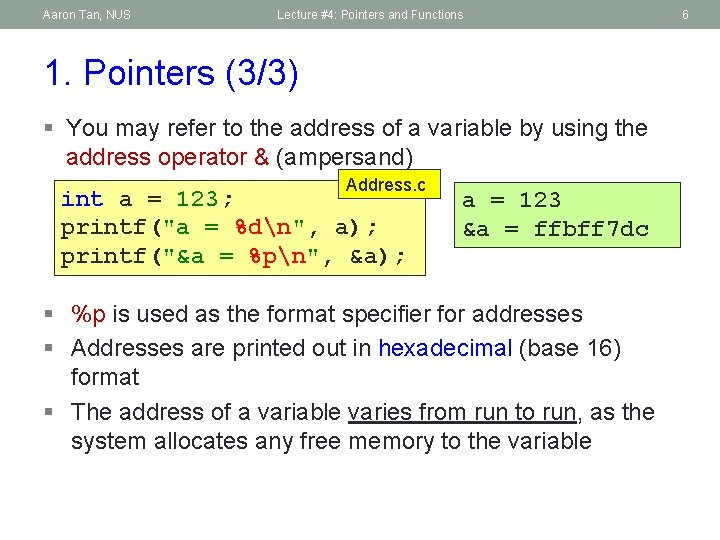Aaron Tan, NUS Lecture #4: Pointers and Functions 1. Pointers (3/3) § You may refer to the address of a variable by using the address operator & (ampersand) Address. c int a = 123; printf("a = %dn", a); printf("&a = %pn", &a); a = 123 &a = ffbff 7 dc § %p is used as the format specifier for addresses § Addresses are printed out in hexadecimal (base 16) format § The address of a variable varies from run to run, as the system allocates any free memory to the variable 6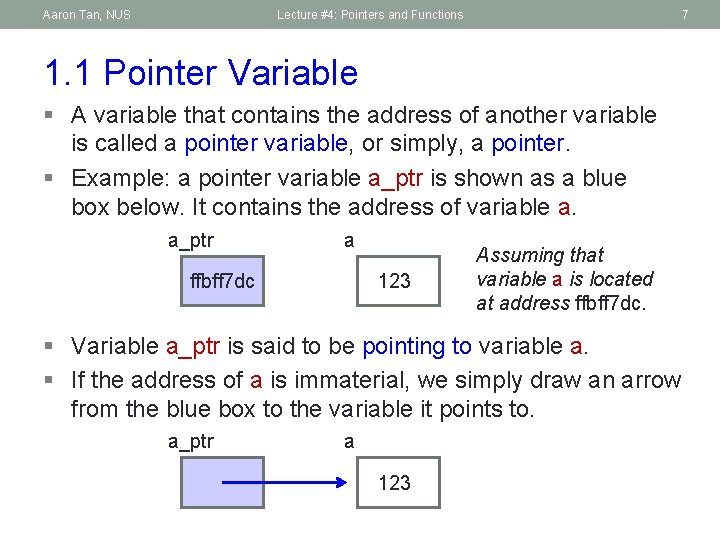Aaron Tan, NUS Lecture #4: Pointers and Functions 7 1. 1 Pointer Variable § A variable that contains the address of another variable is called a pointer variable, or simply, a pointer. § Example: a pointer variable a_ptr is shown as a blue box below. It contains the address of variable a. a_ptr a ffbff 7 dc 123 Assuming that variable a is located at address ffbff 7 dc. § Variable a_ptr is said to be pointing to variable a. § If the address of a is immaterial, we simply draw an arrow from the blue box to the variable it points to. a_ptr a 123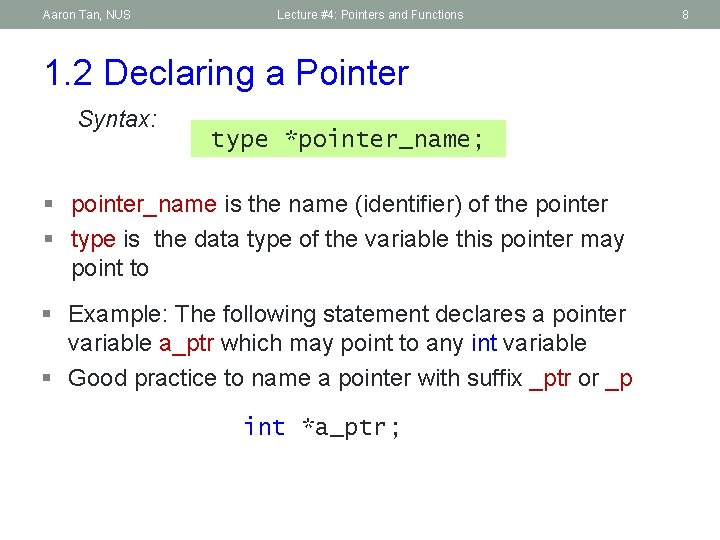Aaron Tan, NUS Lecture #4: Pointers and Functions 1. 2 Declaring a Pointer Syntax: type *pointer_name; § pointer_name is the name (identifier) of the pointer § type is the data type of the variable this pointer may point to § Example: The following statement declares a pointer variable a_ptr which may point to any int variable § Good practice to name a pointer with suffix _ptr or _p int *a_ptr; 8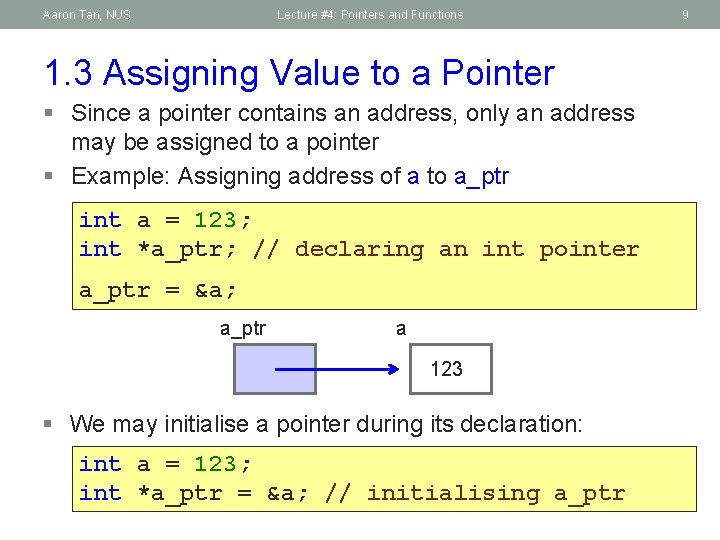Aaron Tan, NUS Lecture #4: Pointers and Functions 1. 3 Assigning Value to a Pointer § Since a pointer contains an address, only an address may be assigned to a pointer § Example: Assigning address of a to a_ptr int a = 123; int *a_ptr; // declaring an int pointer a_ptr = &a; a_ptr a 123 § We may initialise a pointer during its declaration: int a = 123; int *a_ptr = &a; // initialising a_ptr 9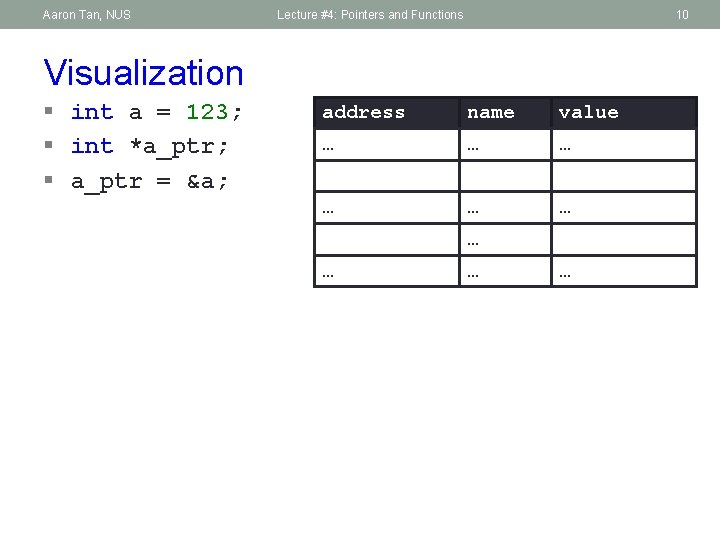Aaron Tan, NUS Lecture #4: Pointers and Functions 10 Visualization § int a = 123; § int *a_ptr; § a_ptr = &a; address name value … … … … …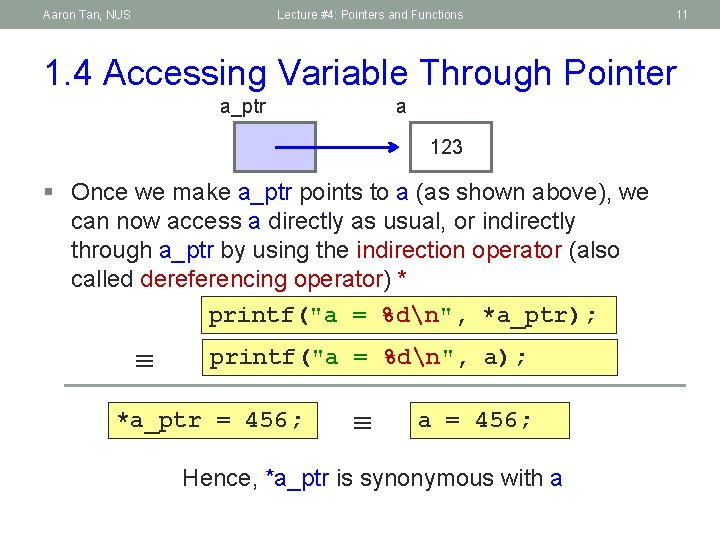Aaron Tan, NUS Lecture #4: Pointers and Functions 11 1. 4 Accessing Variable Through Pointer a_ptr a 123 § Once we make a_ptr points to a (as shown above), we can now access a directly as usual, or indirectly through a_ptr by using the indirection operator (also called dereferencing operator) * printf("a = %dn", *a_ptr); printf("a = %dn", a); *a_ptr = 456; a = 456; Hence, *a_ptr is synonymous with aAaron Tan, NUS Lecture #4: Pointers and Functions 12 1. 5 Example #1 int i = 10, j = 20; int *p; // p is a pointer p = &i; i 12 10 20 to some int variable // p now stores the address of variable i Now *p is equivalent to i printf("value of i is %dn", *p); value of i is 10 // *p accesses the value of pointed/referred variable *p = *p + 2; // increment *p (which is i) by 2 // same effect as: i = i + 2; // p now stores the address of variable j Important! *p = i; 12 p Important! p = &j; j Now *p is equivalent to j // value of *p (which is j now) becomes 12 // same effect as: j = i;Aaron Tan, NUS Lecture #4: Pointers and Functions 13 1. 6 Example #2 (1/2) a Pointer. c #include <stdio. h> int main(void) { double a, *b; Can you draw the picture? What is the output? b = &a; *b = 12. 34; printf("%fn", a); return 0; } b 12. 340000 What is the output if the printf() statement is changed to the following? printf("%fn", *b); printf("%fn", *a); 12. 340000 Compile with warning Error Value in hexadecimal; What is the proper way to print a pointer? varies from run to run. (Seldom need to do this. ) printf("%pn", b); ffbff 6 a 0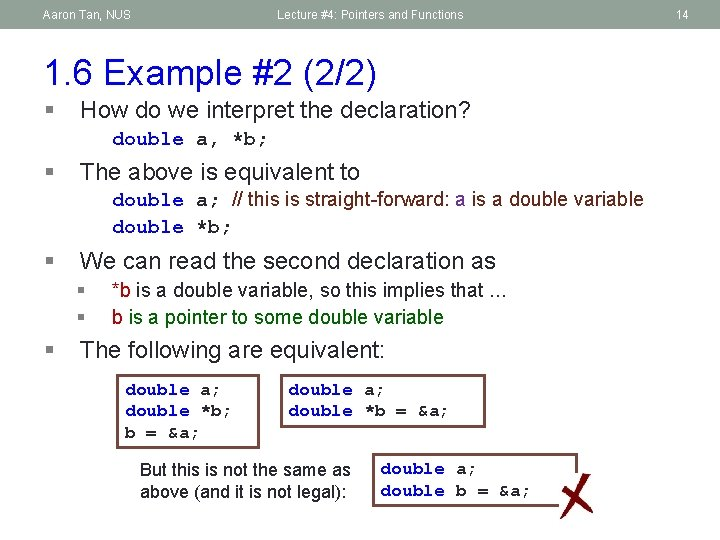Aaron Tan, NUS Lecture #4: Pointers and Functions 1. 6 Example #2 (2/2) § How do we interpret the declaration? double a, *b; § The above is equivalent to double a; // this is straight-forward: a is a double variable double *b; § We can read the second declaration as § § § *b is a double variable, so this implies that. . . b is a pointer to some double variable The following are equivalent: double a; double *b; b = &a; double a; double *b = &a; But this is not the same as above (and it is not legal): double a; double b = &a; 14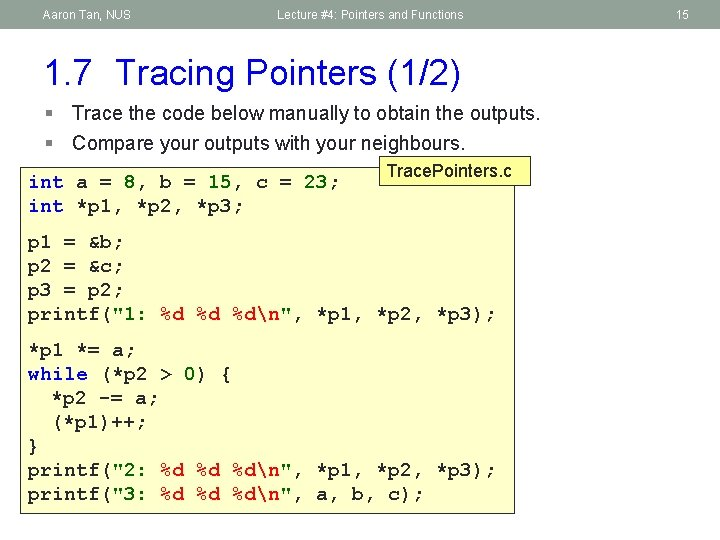Aaron Tan, NUS Lecture #4: Pointers and Functions 1. 7 Tracing Pointers (1/2) § Trace the code below manually to obtain the outputs. § Compare your outputs with your neighbours. int a = 8, b = 15, c = 23; int *p 1, *p 2, *p 3; Trace. Pointers. c p 1 = &b; p 2 = &c; p 3 = p 2; printf("1: %d %d %dn", *p 1, *p 2, *p 3); *p 1 *= a; while (*p 2 > 0) { *p 2 -= a; (*p 1)++; } printf("2: %d %d %dn", *p 1, *p 2, *p 3); printf("3: %d %d %dn", a, b, c); 15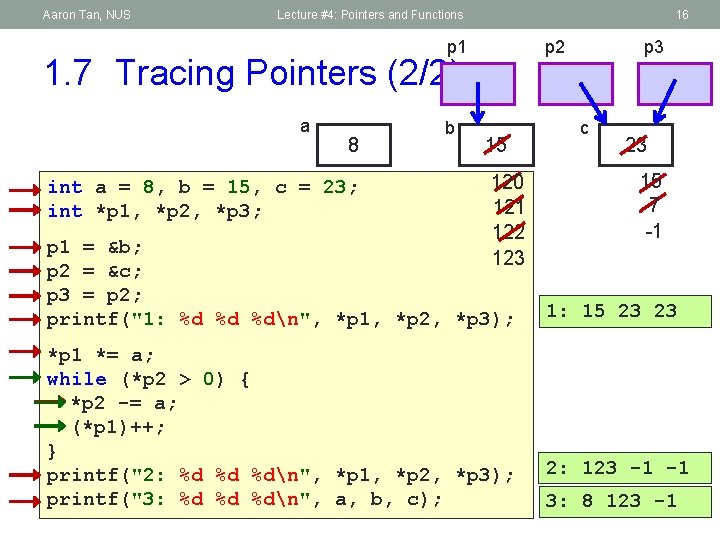Aaron Tan, NUS Lecture #4: Pointers and Functions 16 p 1 p 2 1. 7 Tracing Pointers (2/2) a 8 int a = 8, b = 15, c = 23; int *p 1, *p 2, *p 3; b 15 120 121 122 123 p 1 = &b; p 2 = &c; p 3 = p 2; printf("1: %d %d %dn", *p 1, *p 2, *p 3); *p 1 *= a; while (*p 2 > 0) { *p 2 -= a; (*p 1)++; } printf("2: %d %d %dn", *p 1, *p 2, *p 3); printf("3: %d %d %dn", a, b, c); p 3 c 23 15 7 -1 1: 15 23 23 2: 123 -1 -1 3: 8 123 -1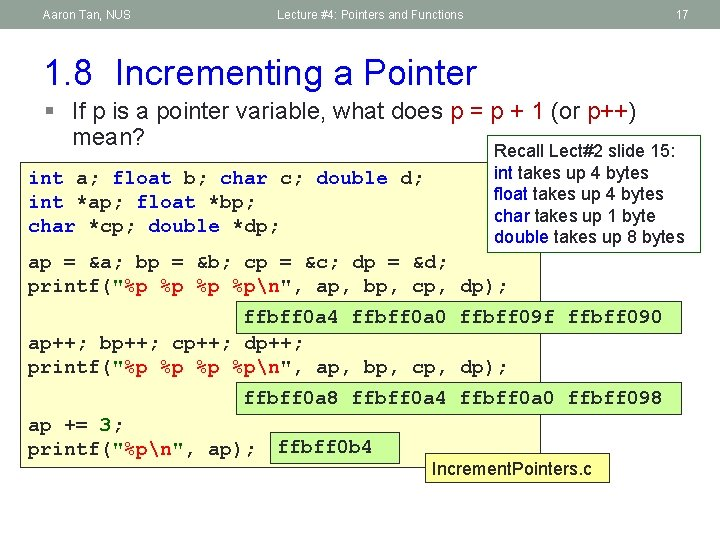Aaron Tan, NUS Lecture #4: Pointers and Functions 17 1. 8 Incrementing a Pointer § If p is a pointer variable, what does p = p + 1 (or p++) mean? Recall Lect#2 slide 15: int a; float b; char c; double d; int *ap; float *bp; char *cp; double *dp; int takes up 4 bytes float takes up 4 bytes char takes up 1 byte double takes up 8 bytes ap = &a; bp = &b; cp = &c; dp = &d; printf("%p %pn", ap, bp, cp, dp); ffbff 0 a 4 ffbff 0 a 0 ffbff 09 f ffbff 090 ap++; bp++; cp++; dp++; printf("%p %pn", ap, bp, cp, dp); ffbff 0 a 8 ffbff 0 a 4 ffbff 0 a 0 ffbff 098 ap += 3; printf("%pn", ap); ffbff 0 b 4 Increment. Pointers. cAaron Tan, NUS Lecture #4: Pointers and Functions 18 1. 9 Common Mistake int *n; Common. Mistake. c *n = 123; printf("%dn", *n); What’s wrong with this? Can you draw the picture? n ? § Where is the pointer n pointing to? § Where is the value 123 assigned to? § Result: Segmentation Fault (core dumped) § Remove the file “core” from your directory. It takes up a lot of space!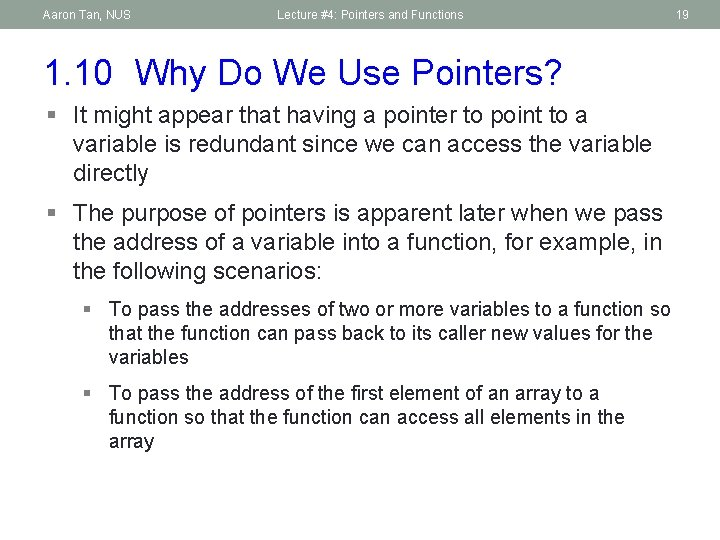Aaron Tan, NUS Lecture #4: Pointers and Functions 1. 10 Why Do We Use Pointers? § It might appear that having a pointer to point to a variable is redundant since we can access the variable directly § The purpose of pointers is apparent later when we pass the address of a variable into a function, for example, in the following scenarios: § To pass the addresses of two or more variables to a function so that the function can pass back to its caller new values for the variables § To pass the address of the first element of an array to a function so that the function can access all elements in the array 19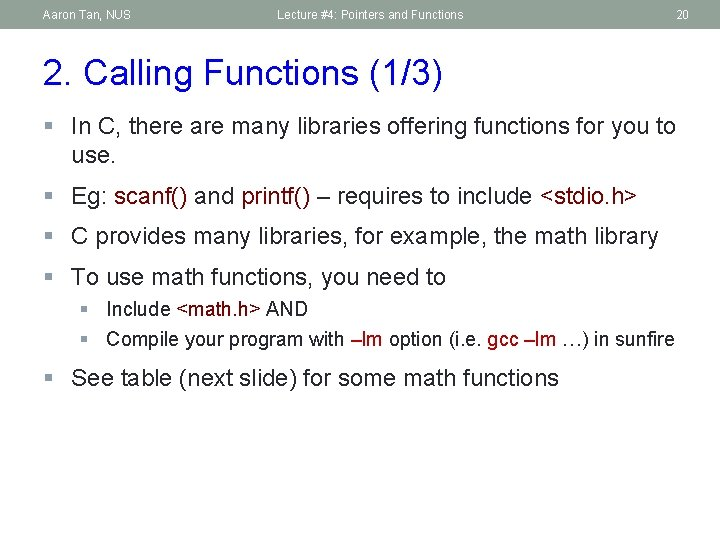Aaron Tan, NUS Lecture #4: Pointers and Functions 20 2. Calling Functions (1/3) § In C, there are many libraries offering functions for you to use. § Eg: scanf() and printf() – requires to include <stdio. h> § C provides many libraries, for example, the math library § To use math functions, you need to § Include <math. h> AND § Compile your program with –lm option (i. e. gcc –lm …) in sunfire § See table (next slide) for some math functionsAaron Tan, NUS Lecture #4: Pointers and Functions 21 2. Calling Functions (2/3) Function prototype: double pow(double x, double y) function return typeAaron Tan, NUS Lecture #4: Pointers and Functions 2. Calling Functions (3/3) #include <stdio. h> #include <math. h> int main(void) { int x, y; float val; Math. Functions. c printf("Enter value: "); scanf("%f", &val); printf("sqrt(%f) = %fn", val, sqrt(val)); } To link to Math library \$ gcc –lm Math. Functions. c \$ a. out Enter x and y: 3 4 pow(3, 4) = 81. 000000 Enter value: 65. 4 sqrt(65. 400002) = 8. 087027 printf("Enter x and y: "); scanf("%d %d", &x, &y); printf("pow(%d, %d) = %fn", x, y, pow(x, y)); return 0; 22Aaron Tan, NUS Lecture #4: Pointers and Functions 3. User-Defined Functions (1/6) § We can define and use our own functions Example: Compute the volume of a flat washer. Dimensions of a flat washer are usually given as an inner diameter, an outer diameter, and a thickness. rim area = (d 2/2)2 – (d 1/2)2 23Aaron Tan, NUS Lecture #4: Pointers and Functions 3. User-Defined Functions (2/6) Washer. c #include <stdio. h> Enter …: 8. 2 10. 5 2. 2 #include <math. h> #define PI 3. 14159 Volume of washer = 74. 32 int main(void) { double d 1, // inner diameter d 2, // outer diameter thickness, outer_area, inner_area, rim_area, volume; // read input data printf("Enter inner diameter, outer diameter, thickness: "); scanf("%lf %lf", &d 1, &d 2, &thickness); // compute volume of a washer outer_area = PI * pow(d 2/2, 2); inner_area = PI * pow(d 1/2, 2); rim_area = outer_area - inner_area; volume = rim_area * thickness; printf("Volume of washer = %. 2 fn", volume); return 0; } 24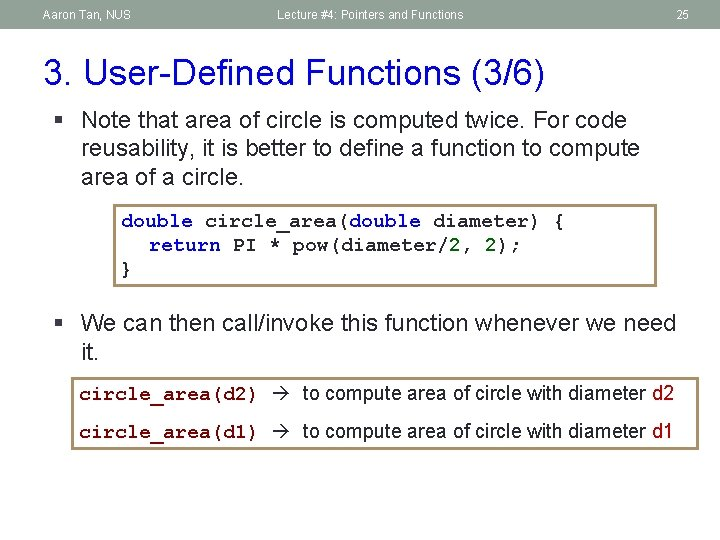Aaron Tan, NUS Lecture #4: Pointers and Functions 25 3. User-Defined Functions (3/6) § Note that area of circle is computed twice. For code reusability, it is better to define a function to compute area of a circle. double circle_area(double diameter) { return PI * pow(diameter/2, 2); } § We can then call/invoke this function whenever we need it. circle_area(d 2) to compute area of circle with diameter d 2 circle_area(d 1) to compute area of circle with diameter d 1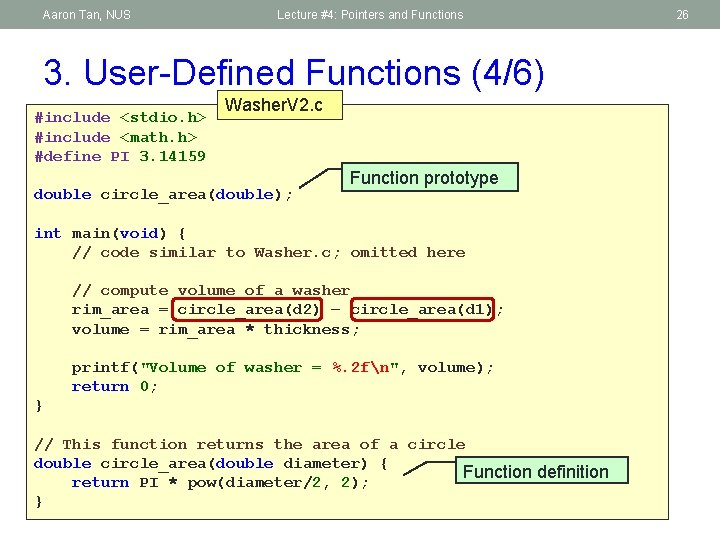Aaron Tan, NUS Lecture #4: Pointers and Functions 3. User-Defined Functions (4/6) #include <stdio. h> #include <math. h> #define PI 3. 14159 Washer. V 2. c double circle_area(double); Function prototype int main(void) { // code similar to Washer. c; omitted here // compute volume of a washer rim_area = circle_area(d 2) – circle_area(d 1); volume = rim_area * thickness; printf("Volume of washer = %. 2 fn", volume); return 0; } // This function returns the area of a circle double circle_area(double diameter) { Function definition return PI * pow(diameter/2, 2); } 26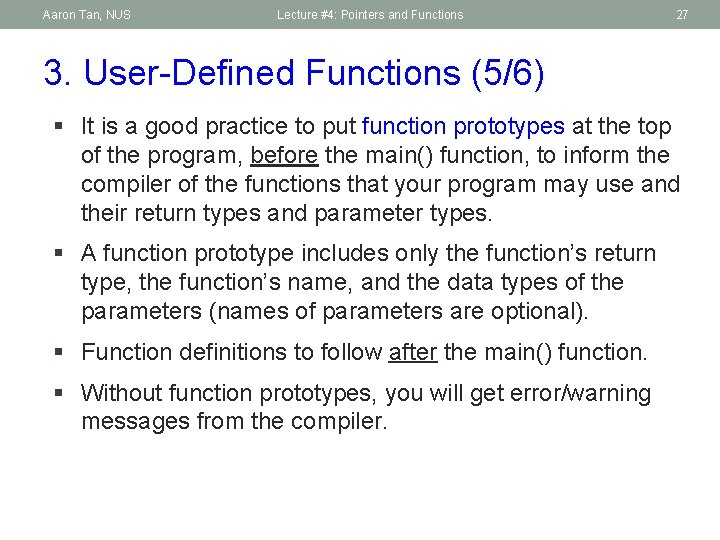Aaron Tan, NUS Lecture #4: Pointers and Functions 27 3. User-Defined Functions (5/6) § It is a good practice to put function prototypes at the top of the program, before the main() function, to inform the compiler of the functions that your program may use and their return types and parameter types. § A function prototype includes only the function’s return type, the function’s name, and the data types of the parameters (names of parameters are optional). § Function definitions to follow after the main() function. § Without function prototypes, you will get error/warning messages from the compiler.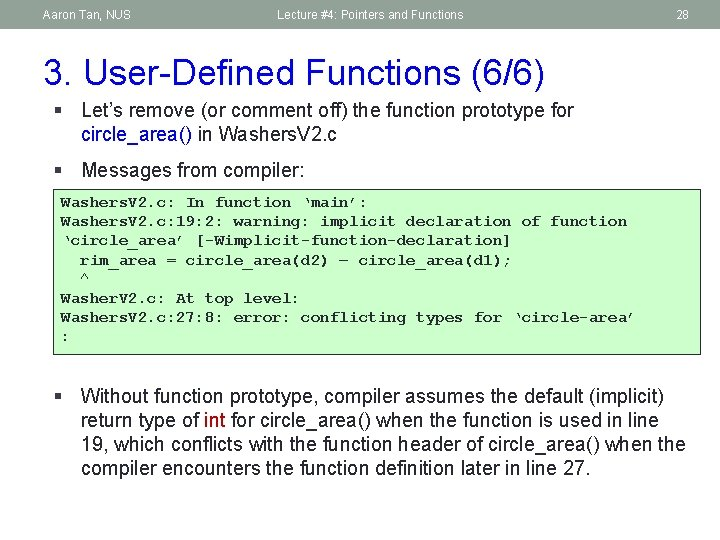Aaron Tan, NUS Lecture #4: Pointers and Functions 28 3. User-Defined Functions (6/6) § Let’s remove (or comment off) the function prototype for circle_area() in Washers. V 2. c § Messages from compiler: Washers. V 2. c: In function ‘main’: Washers. V 2. c: 19: 2: warning: implicit declaration of function ‘circle_area’ [-Wimplicit-function-declaration] rim_area = circle_area(d 2) – circle_area(d 1); ^ Washer. V 2. c: At top level: Washers. V 2. c: 27: 8: error: conflicting types for ‘circle-area’ : § Without function prototype, compiler assumes the default (implicit) return type of int for circle_area() when the function is used in line 19, which conflicts with the function header of circle_area() when the compiler encounters the function definition later in line 27.Aaron Tan, NUS Lecture #4: Pointers and Functions 29 4. Pass-by-Value and Scope Rule (1/4) § In C, the actual parameters are passed to the formal parameters by a mechanism known as pass-by-value. int main(void) { double a = 10. 5, b = 7. 8; printf("%. 2 fn", sqrt_sum_square(3. 2, 12/5); printf("%. 2 fn", sqrt_sum_square(a, a+b); return 0; a b 10. 5 7. 8 Actual parameters: 10. 5 3. 2 and 18. 3 2. 0 } double sqrt_sum_square(double x, double y) { double sum_square; sum_square = pow(x, 2) + pow(y, 2); return sqrt(sum_square); } Formal parameters: x y 10. 5 3. 2 18. 3 2. 0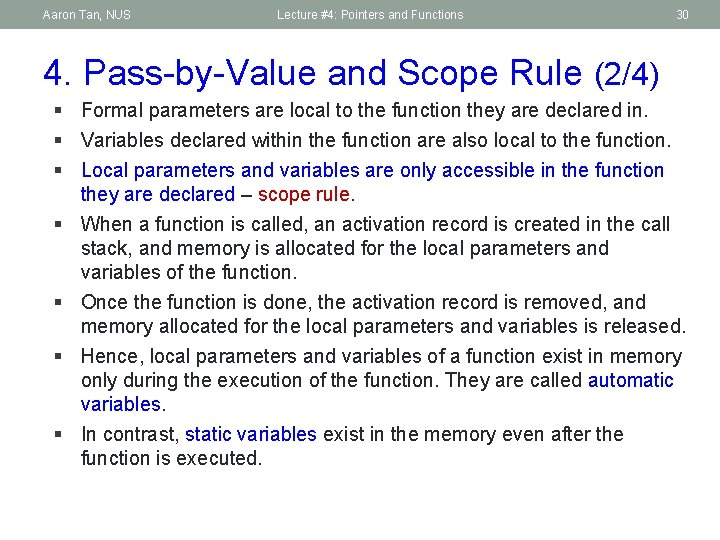Aaron Tan, NUS Lecture #4: Pointers and Functions 30 4. Pass-by-Value and Scope Rule (2/4) § Formal parameters are local to the function they are declared in. § Variables declared within the function are also local to the function. § Local parameters and variables are only accessible in the function they are declared – scope rule. § When a function is called, an activation record is created in the call stack, and memory is allocated for the local parameters and variables of the function. § Once the function is done, the activation record is removed, and memory allocated for the local parameters and variables is released. § Hence, local parameters and variables of a function exist in memory only during the execution of the function. They are called automatic variables. § In contrast, static variables exist in the memory even after the function is executed.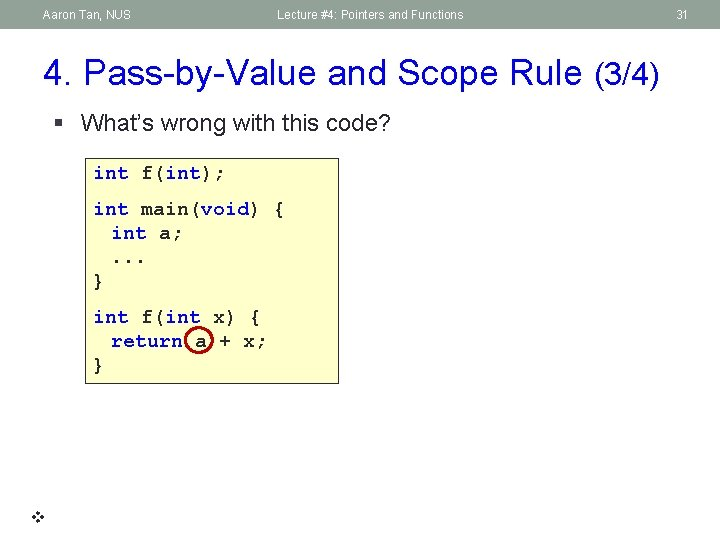Aaron Tan, NUS Lecture #4: Pointers and Functions 4. Pass-by-Value and Scope Rule (3/4) § What’s wrong with this code? int f(int); int main(void) { int a; . . . } int f(int x) { return a + x; } 31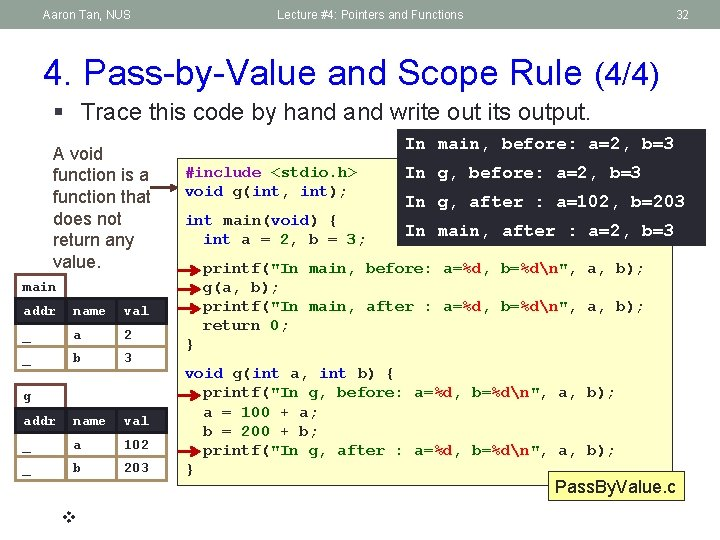Aaron Tan, NUS Lecture #4: Pointers and Functions 32 4. Pass-by-Value and Scope Rule (4/4) § Trace this code by hand write out its output. A void function is a function that does not return any value. In main, before: a=2, b=3 #include <stdio. h> void g(int, int); int main(void) { int a = 2, b = 3; name val _ a 2 _ b 3 addr name val _ a 2 102 _ b 3 203 g In g, after : a=102, b=203 In main, after : a=2, b=3 printf("In main, before: a=%d, b=%dn", a, b); g(a, b); printf("In main, after : a=%d, b=%dn", a, b); return 0; main addr In g, before: a=2, b=3 } void g(int a, int b) { printf("In g, before: a=%d, b=%dn", a, b); a = 100 + a; b = 200 + b; printf("In g, after : a=%d, b=%dn", a, b); } Pass. By. Value. c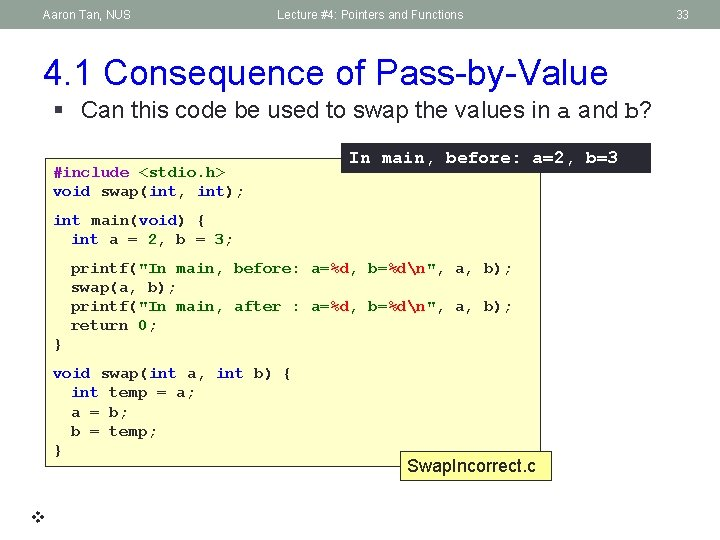Aaron Tan, NUS Lecture #4: Pointers and Functions 4. 1 Consequence of Pass-by-Value § Can this code be used to swap the values in a and b? #include <stdio. h> void swap(int, int); In main, before: a=2, b=3 int main(void) { int a = 2, b = 3; printf("In main, before: a=%d, b=%dn", a, b); swap(a, b); printf("In main, after : a=%d, b=%dn", a, b); return 0; } void swap(int a, int b) { int temp = a; a = b; b = temp; } Swap. Incorrect. c 33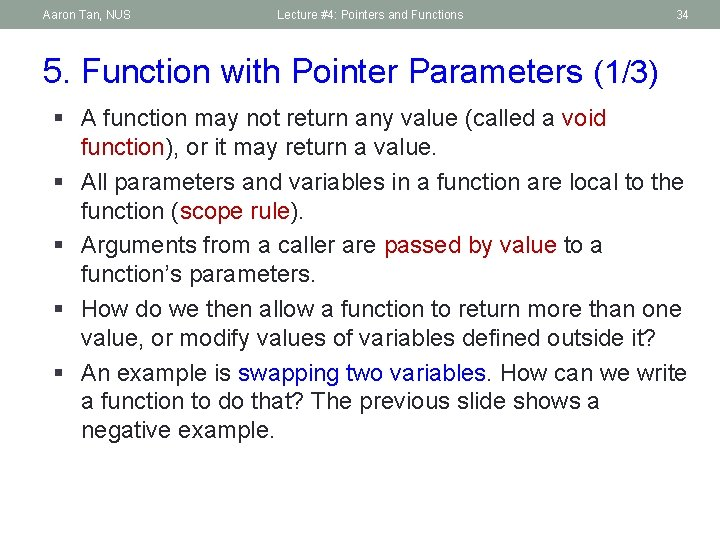Aaron Tan, NUS Lecture #4: Pointers and Functions 34 5. Function with Pointer Parameters (1/3) § A function may not return any value (called a void function), or it may return a value. § All parameters and variables in a function are local to the function (scope rule). § Arguments from a caller are passed by value to a function’s parameters. § How do we then allow a function to return more than one value, or modify values of variables defined outside it? § An example is swapping two variables. How can we write a function to do that? The previous slide shows a negative example.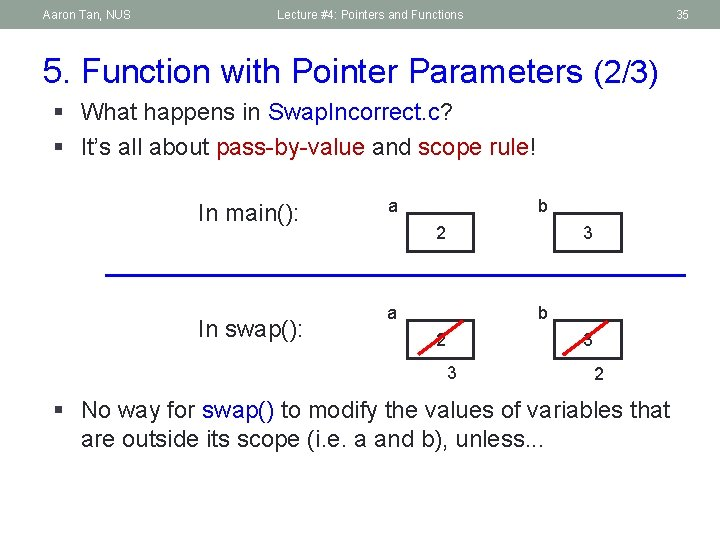Aaron Tan, NUS Lecture #4: Pointers and Functions 35 5. Function with Pointer Parameters (2/3) § What happens in Swap. Incorrect. c? § It’s all about pass-by-value and scope rule! In main(): In swap(): a b 2 3 3 2 § No way for swap() to modify the values of variables that are outside its scope (i. e. a and b), unless. . .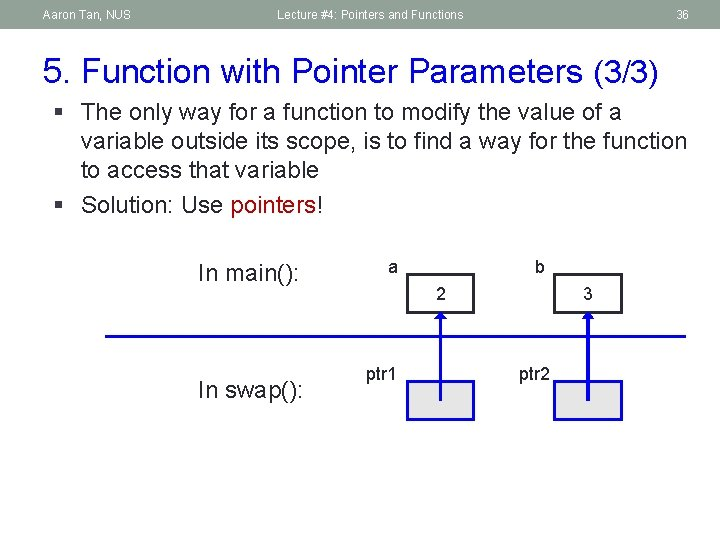Aaron Tan, NUS Lecture #4: Pointers and Functions 36 5. Function with Pointer Parameters (3/3) § The only way for a function to modify the value of a variable outside its scope, is to find a way for the function to access that variable § Solution: Use pointers! In main(): In swap(): a b 2 ptr 1 3 ptr 2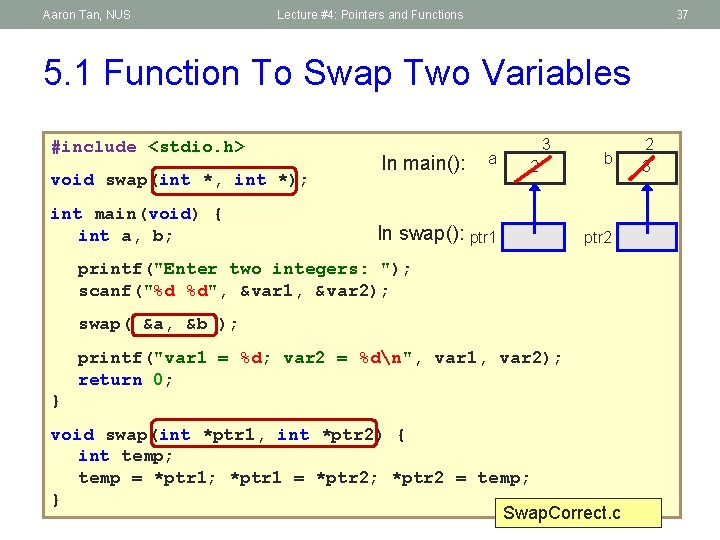Aaron Tan, NUS Lecture #4: Pointers and Functions 37 5. 1 Function To Swap Two Variables #include <stdio. h> void swap(int *, int *); int main(void) { int a, b; In main(): a 3 2 In swap(): ptr 1 b ptr 2 printf("Enter two integers: "); scanf("%d %d", &var 1, &var 2); swap( &a, &b ); printf("var 1 = %d; var 2 = %dn", var 1, var 2); return 0; } void swap(int *ptr 1, int *ptr 2) { int temp; temp = *ptr 1; *ptr 1 = *ptr 2; *ptr 2 = temp; } Swap. Correct. c 2 3Aaron Tan, NUS Lecture #4: Pointers and Functions 38 5. 2 Examples (1/4) #include <stdio. h> void f(int, int); Example 1. c a b c int main(void) { 9 -2 int a = 9, b = -2, c = 5; f(a, b, c); printf("a = %d, b = %d, c = %dn", a, b, c); return 0; } x y z void f(int x, int y, int z) { 9 -2 x = 3 + y; 1 10 y = 10 * x; z = x + y + z; printf("x = %d, y = %d, z = %dn", x, y, z); } 5 5 16 x = 1, y = 10, z = 16 a = 9, b = -2, c = 5Aaron Tan, NUS Lecture #4: Pointers and Functions 5. 2 Examples (2/4) #include <stdio. h> void f(int *, int *); 39 Example 2. c 1 10 int main(void) { a b c 9 -2 int a = 9, b = -2, c = 5; f(&a, &b, &c); printf("a = %d, b = %d, c = %dn", a, b, c); return 0; } 16 5 y z void f(int *x, int *y, int *z) x { *x = 3 + *y; *x is a, *y is b, and *y = 10 * *x; *z = *x + *y + *z; printf("*x = %d, *y = %d, *z = %dn", *x, *y, *z); } *x = 1, *y = 10, *z = 16 a = 1, b = 10, c = 16 *z is c!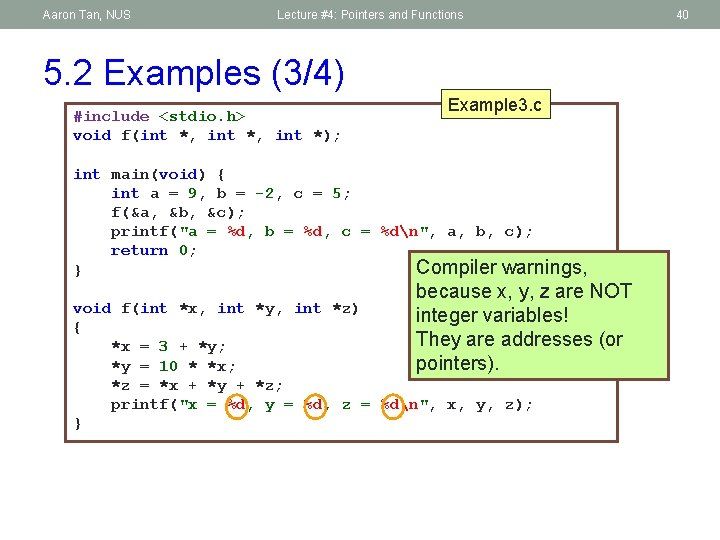Aaron Tan, NUS Lecture #4: Pointers and Functions 5. 2 Examples (3/4) #include <stdio. h> void f(int *, int *); Example 3. c int main(void) { int a = 9, b = -2, c = 5; f(&a, &b, &c); printf("a = %d, b = %d, c = %dn", a, b, c); return 0; Compiler warnings, } because x, y, z are NOT integer variables! They are addresses (or pointers). void f(int *x, int *y, int *z) { *x = 3 + *y; *y = 10 * *x; *z = *x + *y + *z; printf("x = %d, y = %d, z = %dn", x, y, z); } 40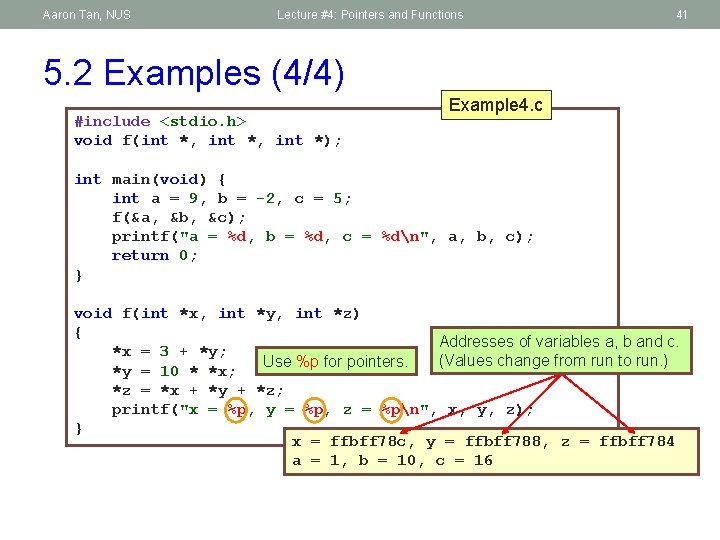Aaron Tan, NUS Lecture #4: Pointers and Functions 41 5. 2 Examples (4/4) #include <stdio. h> void f(int *, int *); Example 4. c int main(void) { int a = 9, b = -2, c = 5; f(&a, &b, &c); printf("a = %d, b = %d, c = %dn", a, b, c); return 0; } void f(int *x, int *y, int *z) { Addresses of variables a, b and c. *x = 3 + *y; (Values change from run to run. ) Use %p for pointers. *y = 10 * *x; *z = *x + *y + *z; printf("x = %p, y = %p, z = %pn", x, y, z); } x = ffbff 78 c, y = ffbff 788, z = ffbff 784 a = 1, b = 10, c = 16Aaron Tan, NUS Lecture #4: Pointers and Functions End of File 42